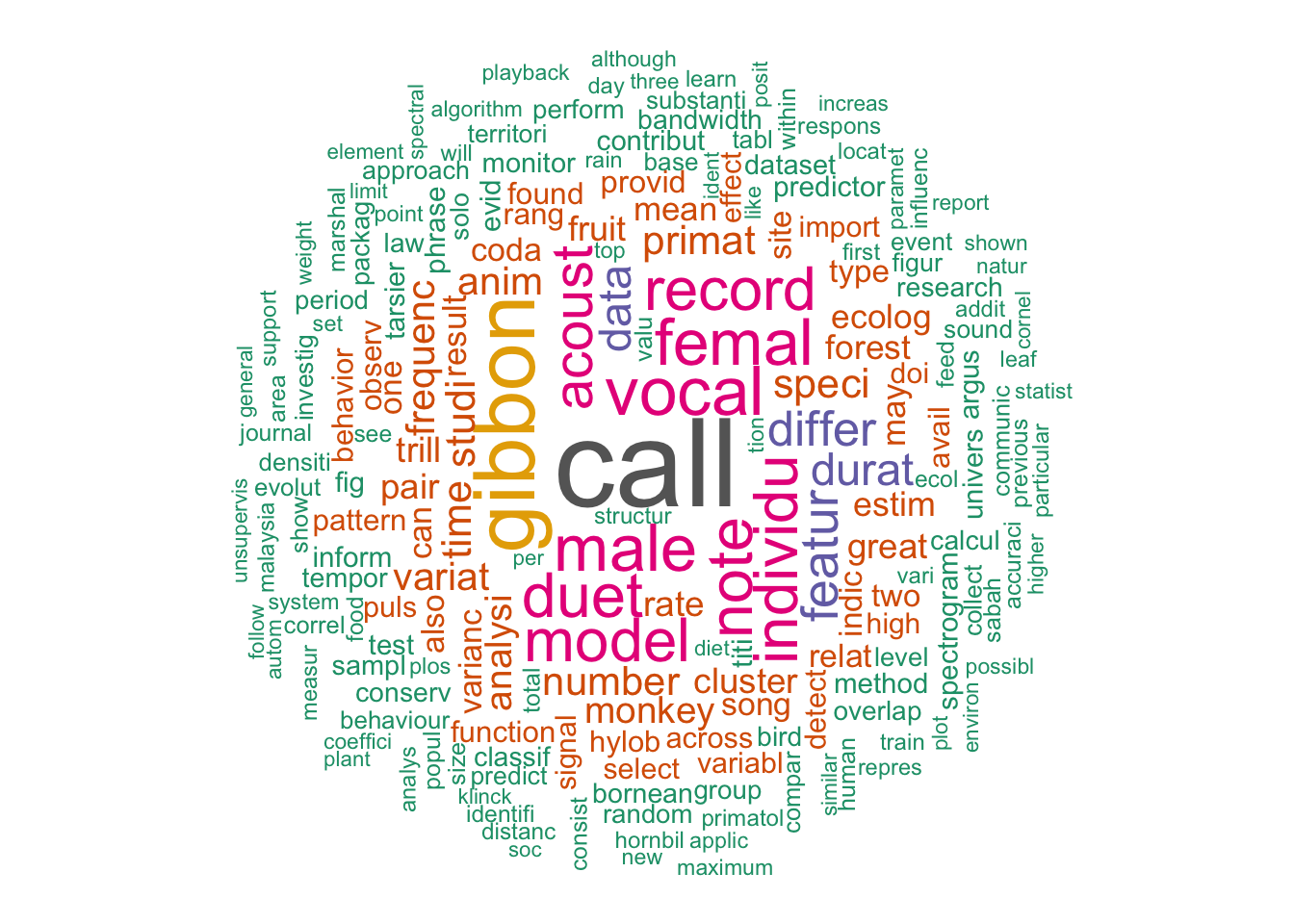# Creating a wordcloud using R!

Here I create a word cloud from my publications list.Figure 1: A word cloud from my current publications

### Overview

Wordclouds can be a great way to identify recurring themes in documents.

### Wordcloud tutorial

First you need to load the relevant libraries.

``````library(pdftools)
library(wordcloud)
library(RColorBrewer)
library(wordcloud2)
library(tm)
library(dplyr)``````

Then you tell R where the folder with the PDFs you want to use are located.

``files <- list.files("/Users/denaclink/Desktop/Clink Publications /",full.names = T)``

We then use the ‘Corpus’ function to extract text from the PDF documents.

``````corp <- Corpus(URISource(files),
print(corp)
``````

We can then create a document-term matrix that describes the frequency of terms that occur in the documents.

``````publications.tdm <- TermDocumentMatrix(corp,
control =
list(removePunctuation = TRUE,
stopwords = TRUE,
tolower = TRUE,
stemming = TRUE,
removeNumbers = TRUE,
bounds = list(global = c(3, Inf)))) ``````

We can then do some data processing to prepare to input the document-term matrix to the wordcloud.

``````# Convert the output to a matrix
matrix <- as.matrix(publications.tdm)

# We then count the frequency of the use of different words
words <- sort(rowSums(matrix),decreasing=TRUE)

# Convert that output to a dataframe
df <- data.frame(word = names(words),freq=words)

# Remove words that we don't want to include in the wordcloud
remove.rows <- which(df\$word %in% c('clink','use','includ') )
df <- df[- remove.rows,]``````

Then we use the ‘wordcloud’ function to create our wordcloud!

``````wordcloud(words = df\$word, freq = df\$freq, min.freq = 4,
max.words=200, random.order=FALSE, rot.per=0.35,
colors=brewer.pal(8, "Dark2"))``````